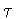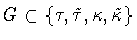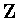Next: Discrete Corkscrew Columns Up: Columns with Discrete Symmetry Previous: Columns with Discrete Symmetry

### Periodic Columns with No Corkscrew Symmetry -- Eight Types

Recall thatis a reflection through a plane containing the axis of the cylinder andis the reflection through the midplane - the up-down symmetry. Each of these symmetries has a glide reflectionversionThere are ten subsetsthat form symmetry groups when coupled with.These subsets are:The symmetry groups of the corresponding periodic columns are:--the group generated by G and.Examples of columns having one purereflection symmetry are found in Figures  10and 11. Examples of columns having precisely one glide reflection are given inFigures  12 and 13. Columns having two reflections or glidereflections are shown in Figures  14,15, 16 and 17. The last two subsets correspond to symmetry groups that lie in infinite families and these infinite families have corkscrew symmetries (see Figures  21and 22).Next: Discrete Corkscrew Columns Up: Columns with Discrete Symmetry Previous: Columns with Discrete Symmetry
Marty Golubitsky
2001-01-29